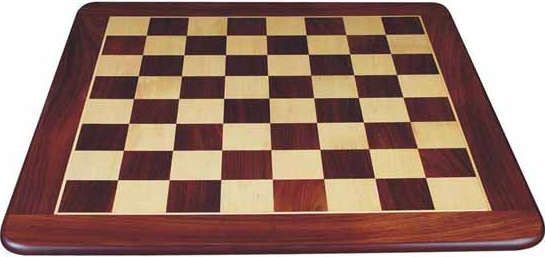# You're all set, diagonally!

Probability Level 3If three $1\times1$ squares are randomly chosen from a standard $8\times8$ chessboard, the probability that they all lie on the same diagonal is $\frac ab$ for coprime positive integers, find the value of $a+b$.

×